# Dirichlet eigenvalue

Consider a bounded domainwith a piecewise smooth boundary.is a Dirichlet eigenvalue ofif there exists a function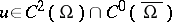(a Dirichlet eigenfunction) satisfying the following Dirichlet boundary value problem (cf. also Dirichlet boundary conditions):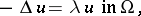(a1)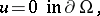(a2)

whereis the Laplace operator (i.e.,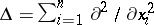). Dirichlet eigenvalues (with) were introduced in the study of the vibrations of the clamped membrane in the nineteenth century. In fact, they are proportional to the square of the eigenfrequencies of the membrane with fixed boundary. See [a9] for a review and historical remarks. Providedis bounded and the boundaryis sufficiently regular, the Dirichlet Laplacian has a discrete spectrum of infinitely many positive eigenvalues with no finite accumulation point [a15]:(a3)

(as).

The Dirichlet eigenvalues are characterized by the max-min principle [a4]:(a4)

where the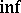is taken over all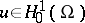orthogonal to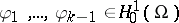, and the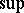is taken over all choices of. For simply-connected domains it follows from the max-min principle (a4) that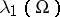is non-degenerate and the corresponding eigenfunction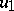is positive in the interior of. For higher values ofthe nodal lines of theth eigenfunction divideinto no more thansubregions (nodal domains; this is Courant's nodal line theorem [a4]). Along this subject, notice the proof of A.D. Melas [a11] of the nodal line conjecture for plane domains (ifis a bounded, smooth, convex domain, the nodal line ofalways meets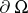).

## Weyl asymptotics.

For large values of, if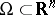, H. Weyl [a17], [a18] proved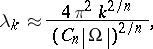(a5)

where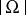and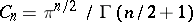are, respectively, the volumes ofand of the unit ball in.

## Pólya conjecture.

For any plane-covering domain (i.e., a domain that can be used to tile the plane without gaps, nor overlaps, allowing rotations, translations and reflections of itself), G. Pólya [a13] proved that(a6)

and conjectured the same bound for any bounded domain in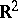(hereis the area of the domain). Pólya's conjecture indimensions is equivalent to saying that the Weyl asymptotics of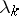, (a5), is a lower bound for, i.e.,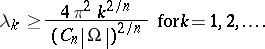(a7)

A result analogous to (a6) for the Neumann eigenvalues of tiling domains, with the sign of the equalities reversed, also holds (cf. also Neumann eigenvalue). The best result to date (2000) towards the proof of the Pólya conjecture is the bound [a10]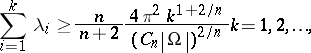(a8)

proven using the asymptotic behaviour of the heat kernel of(cf. also Heat equation) and the connection between the heat kernel and the Dirichlet eigenvalues of a domain (see, e.g., [a6] for a review and related results).

## Kac problem.

Dirichlet eigenvalues are completely characterized by the geometry of the domain. The inverse problem, i.e., up to what extent the geometry ofcan be recovered from the knowledge of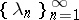, was posed by M. Kac in [a8]. If, for example, andis smooth (in particulardoes not have corners), then the distribution function behaves as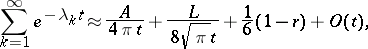(a9)

as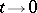, whereis the area,the perimeter andthe number of holes of, so at least these features of the domain can be recovered from knowledge of all the eigenvalues (the first term in (a9) is just a consequence of Weyl's asymptotics). However, complete recovery of the geometry is impossible, as was later shown by C. Gordon, D. Web and S. Wolpert, who constructed two isospectral domains inwith different geometries [a7].

## Eigenvalues and geometry.

The inverse of the square root of a Dirichlet eigenvalue is a length that may be compared with other characteristic lengths of the domain. A typical such comparison is the Rayleigh–Faber–Krahn inequality. Another inequality along these lines is the following: Ifis a simply connected domain inand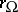is the radius of the largest disc contained in, then there is a universal constantsuch that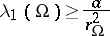(a10)

(as of 2000, the best, not yet optimal, constant in (a10) is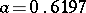; see [a2] for details and historical facts). For other isoperimetric inequalities, see, e.g., [a1], [a12], [a14]. In the same vein, one can also compare Dirichlet and Neumann eigenvalues (see Neumann eigenvalue).

Because of the connection between potential theory and Brownian motion, it is possible to use probabilistic methods to find properties of Dirichlet eigenvalues. One such property was found by H. Brascamp and E.H. Lieb [a3] for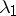: Ifand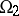are domains in, and one sets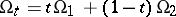, then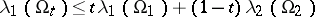for all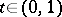. Another example of the use of probabilistic methods is the proof of (a10) by R. Bañuelos and T. Carroll [a2].

To conclude, note that it is possible to define Dirichlet eigenvalues for much more general domains in(see, e.g., [a16], p. 263), and also for the Laplace–Beltrami operator defined on domains in Riemannian manifolds (see, e.g., [a5]).